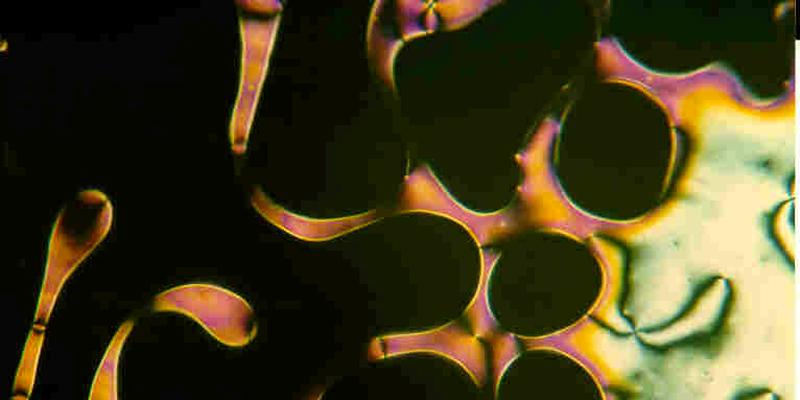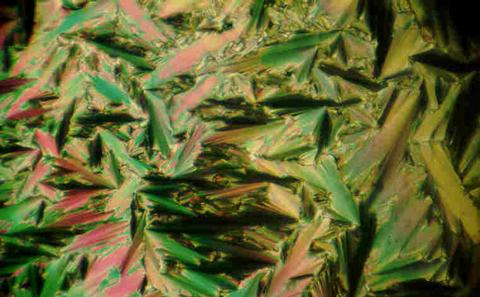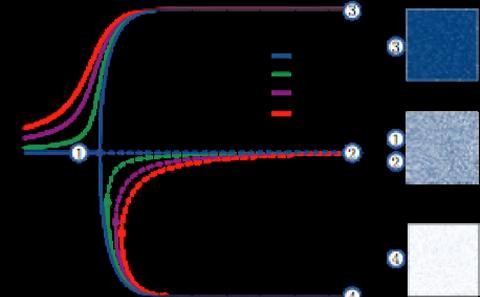The University of Southampton
Mathematical Sciences

# Research project: Computational Multiscale Modelling

Currently Active:
Yes

We model materials and devices, such as liquid crystals and solar cells, which have structures on different length scales. For example, an electric battery can be described on three scales: atomic (chemical reactions), mesoscopic (electrode structure) and macroscopic (global current flow). To model such systems, we develop and use powerful computational and mathematical tools, ranging from a range of PDE solvers to equation-free methods.## Liquid crystals

Liquid C crystals are a different state of matter which exhibit a degree of order between that of a fluid and a solid. Read more below...## Equation-free methods

Equation-free methods provide a bridge between microscopic descriptions of stochastic systems and macroscopic viewpoints of the same system. Read more below...

### Liquid crystals

Physics textbooks normally identify as the three phases of matter, the solid, liquid and vapour phases. Liquid C crystals are a different state of matter which exhibit a degree of order between that of a fluid and a solid: its molecules are not in a fixed position, as is the case of liquids, but may have very similar orientations, as is the case of crystals. Liquid crystals have attracted interest for many reasons: the more widespread is their use in displays, but they are also used as optical light modulator and sensors; many biological materials for liquid crystalline phases; last, but certainly not least, their study gives rise to much interesting mathematics, from broken symmetries and group theory to novel numerical methods. Our interests in this field span from the analysis of their symmetries, to the statistical physics of their phases, the numerical calculation of their alignment and modelling their response in real world devices. This last work is in collaboration with the Functional Materials group in Physics and Astronomy.

### Equation-free methods

Equation-free methods provide a bridge between microscopic descriptions of stochastic systems such as individual-based models, and macroscopic viewpoints of the same system, for example population-level perspectives. They not only allow for accelerated simulation at the macroscopic level but also enable system-level tasks, such as macroscopic bifurcation analysis. In the equation-free framework, we assume the existence of a closed macroscopic model in terms of a few state variables. However, instead of deriving an approximate macroscopic model analytically, we construct a computational superstructure, wrapped around a microscopic simulation. We have applied equation-free methods to understanding switching in two-component system signalling in bacteria, and have also developed improvements to the efficiency of the equation-free algorithm in the context of opinion dynamics.

### Related research groups

Applied Mathematics and Theoretical Physics
Computational Applied Mathematics